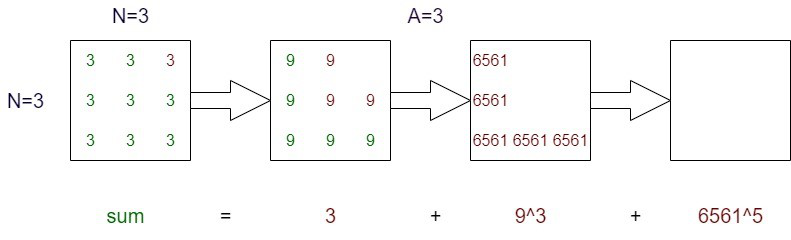GeeksforGeeks App
Open AppBrowser
Continue

# Calculate sum in Diagonal Matrix Decomposition by removing elements in L-shape

Given two integers N representing the dimension of a square matrix and an integer A with which the matrix is initialized. Given another integer mod. Calculate the required sum by following the given steps:

• Select the product of all elements in L shape beginning from the topmost right element, add it to the sum and remove all of them from the matrix.
• Multiply the term removed in the previous step with every other element remaining in the matrix.
• As the sum may be very large, print it modulo mod.

Examples:

Input: N = 3, A = 3, mod = 1000000007
Output: 953271922
Explanation:  1.2157665459E19 % 1000000007 = 953271922Calculate sum in Diagonal Matrix Decomposition by removing elements in L-shape

Input: N = 2, A = 1, mod = 2
Output: 0

Approach: It is obvious that matrices for big dimensions cannot be created. Also, it can be observed that a series is being formed with odd powers on every term where every term has base as one more power of previous term and exponent as number of elements being removed every time. Follow the given steps to solve the problem:

• Create a fast Modular Exponentiation function Mod_Power using the concept of Binary Exponentiation.
• Pass A, 2*i-1, and mod to Mod_Power where 2*i-1 are the odd powers starting from 1 and store the result in(say in a variable term).
• Compute sum by adding all values of term.
• Update base of new term A as product of term and A.

Below is the implementation of the above approach.

## C++14

 `// C++ code to implement the approach``#include ``using` `namespace` `std;``typedef` `long` `long` `ll;` `// Function to calculate the power``ll Mod_Power(ll x, ll y, ll m)``{``    ``ll res = 1;` `    ``while` `(y) {``        ``if` `(y & 1)``            ``res = (res * x) % m;``        ``x = ((x * x) % m + m) % m;``        ``y = y >> 1; ``// y=y/2``    ``}``    ``return` `(res % m + m) % m;``}` `// Function to get the required sum``ll req_Sum(ll n, ll a, ll m)``{``    ``ll sum = 0, term;` `    ``for` `(``int` `i = 1; i <= n; i++) {``        ``term = Mod_Power(a, 2 * i - 1, m);``        ``sum += (term % m);``        ``a = ((a * term) % m + m) % m;``    ``}``    ``return` `(sum % m + m) % m;``}` `// driver's code``int` `main()``{``    ``int` `N = 3;``    ``int` `A = 3;``    ``int` `mod = 1000000007;``    ``cout << req_Sum(N, A, mod);` `    ``return` `0;``}``// this code is contributed by prophet1999`

## Java

 `import` `java.util.*;` `public` `class` `GFG {``    ``// Function to calculate the power``    ``static` `long` `Mod_Power(``long` `x, ``long` `y, ``long` `mod)``    ``{``        ``long` `res = ``1``;``        ``while` `(y > ``0``) {``            ``if` `(y % ``2` `== ``1``)``                ``res = (res * x) % mod;``            ``x = ((x * x) % mod + mod) % mod;``            ``y = y >> ``1``;``        ``}``        ``return` `(res % mod + mod) % mod;``    ``}` `    ``// Function to get the required sum``    ``static` `long` `req_Sum(``long` `N, ``long` `A, ``long` `mod)``    ``{``        ``long` `sum = ``0``, term;``        ``for` `(``int` `i = ``1``; i <= N; i++) {``            ``term = Mod_Power(A, ``2` `* i - ``1``, mod);``            ``sum += (term % mod);``            ``A = ((A * term) % mod + mod) % mod;``        ``}``        ``return` `(sum % mod + mod) % mod;``    ``}` `    ``// Driver's code``    ``public` `static` `void` `main(String[] args)``    ``{` `        ``// Java code to implement the approach``        ``int` `N = ``3``;``        ``int` `A = ``3``;``        ``int` `mod = ``1000000007``;``        ``System.out.print(req_Sum(N, A, mod));``    ``}``}``// this code is contributed by prophet1999`

## Python

 `# Python code to implement the approach` `# Function to calculate the power``def` `Mod_Power(x, y, m):``    ` `    ``res ``=` `1` `    ``while` `(y):``        ``if` `(y & ``1``):``            ``res ``=` `(res ``*` `x) ``%` `m``        ``x ``=` `((x ``*` `x) ``%` `m ``+` `m) ``%` `m``        ``y ``=` `y >> ``1` `# y=y/2``    ` `    ``return` `(res ``%` `m ``+` `m) ``%` `m` `# Function to get the required sum``def` `req_Sum(n, a, m):` `    ``sum` `=` `0``    ``term ``=` `0` `    ``for` `i ``in` `range``(``1``, n ``+` `1``):``        ``term ``=` `Mod_Power(a, ``2` `*` `i ``-` `1``, m)``        ``sum` `+``=` `(term ``%` `m)``        ``a ``=` `((a ``*` `term) ``%` `m ``+` `m) ``%` `m``    ` `    ``return` `(``sum` `%` `m ``+` `m) ``%` `m` `# driver's code` `N ``=` `3``A ``=` `3``mod ``=` `1000000007``print``(req_Sum(N, A, mod))` `# this code is contributed by Samim Hossain Mondal.`

## C#

 `// C# code to implement the approach``using` `System;``class` `GFG``{` `  ``// Function to calculate the power``  ``static` `long` `Mod_Power(``long` `x, ``long` `y, ``long` `m)``  ``{``    ``long` `res = 1;` `    ``while` `(y != 0) {``      ``if` `(y % 2 == 1)``        ``res = (res * x) % m;``      ``x = ((x * x) % m + m) % m;``      ``y = y >> 1; ``// y=y/2``    ``}``    ``return` `(res % m + m) % m;``  ``}` `  ``// Function to get the required sum``  ``static` `long` `req_Sum(``long` `n, ``long` `a, ``long` `m)``  ``{``    ``long` `sum = 0, term;` `    ``for` `(``int` `i = 1; i <= n; i++) {``      ``term = Mod_Power(a, 2 * i - 1, m);``      ``sum += (term % m);``      ``a = ((a * term) % m + m) % m;``    ``}``    ``return` `(sum % m + m) % m;``  ``}` `  ``// driver's code``  ``public` `static` `int` `Main()``  ``{``    ``int` `N = 3;``    ``int` `A = 3;``    ``int` `mod = 1000000007;``    ``Console.Write(req_Sum(N, A, mod));``    ``return` `0;``  ``}``}` `// This code is contributed by Taranpreet`

## Javascript

 ``

## PHP

 `> 1;``    ``}``    ``return` `(``\$res` `% ``\$m` `+ ``\$m``) % ``\$m``;``}` `function` `req_Sum(``\$n``, ``\$a``, ``\$m``) {``    ``\$sum` `= 0;``    ``\$term``;``    ``for` `(``\$i` `= 1; ``\$i` `<= ``\$n``; ``\$i``++) {``        ``\$term` `= Mod_Power(``\$a``, 2 * ``\$i` `- 1, ``\$m``);``        ``\$sum` `+= (``\$term` `% ``\$m``);``        ``\$a` `= ((``\$a` `* ``\$term``) % ``\$m` `+ ``\$m``) % ``\$m``;``    ``}``    ``return` `(``\$sum` `% ``\$m` `+ ``\$m``) % ``\$m``;``}` `\$N` `= 3;``\$A` `= 3;``\$mod` `= 1000000007;``echo` `req_Sum(``\$N``, ``\$A``, ``\$mod``);`

Output

`953271922`

Time Complexity: O(N * log (N2))
Auxiliary Space: O(1)

My Personal Notes arrow_drop_up